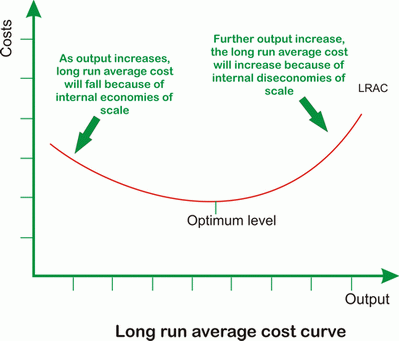# Production in the Long run

In the long run there are no fixed factors of production. All factors are variable, and firms are therefore able to adjust their input of all factors of production. The process of change of input of all factors of production is the change in the scale of production.

When a firm changes it inputs but the change in output is more than the change input, the firms is said to be experiencing increasing returns to scale. On the other hand, when a firm’s output experiences a less proportionate increase as compared to the increase in input, it is said to be diminishing returns to scale.

A firm experiencing an increased return to scale will find its average costs falling. This is termed as economies of scale. Whereas, a rise in average cost of production due to diminishing return to scale are termed as diseconomies of scale.

# Long run cost curves

In the long run all the factors of production are Variable and a firm can expand or decrease the level of output by varying its variable factors.

There is no time dimension as to determine whether it is short run or long run. When the firm can alter it fixed factors, it is said to be a long run.

## Long run Total Cost (LRTC)

It is the total cost incurred as a result of producing a commodity in the long run. It can start from 0 as there is not fixed cost.
Long run total cost will initially increase at a decreasing rate and then at an increasing rate due to law of return to scale.## Long run Average Cost (LRAC)

It is U shaped. In the long run, as output increase, the average cost will fall due to internal economies of scale. As output increases further, average cost will remain constant and if the output is increased further, the average cost will increase again due to internal diseconomies of scale.